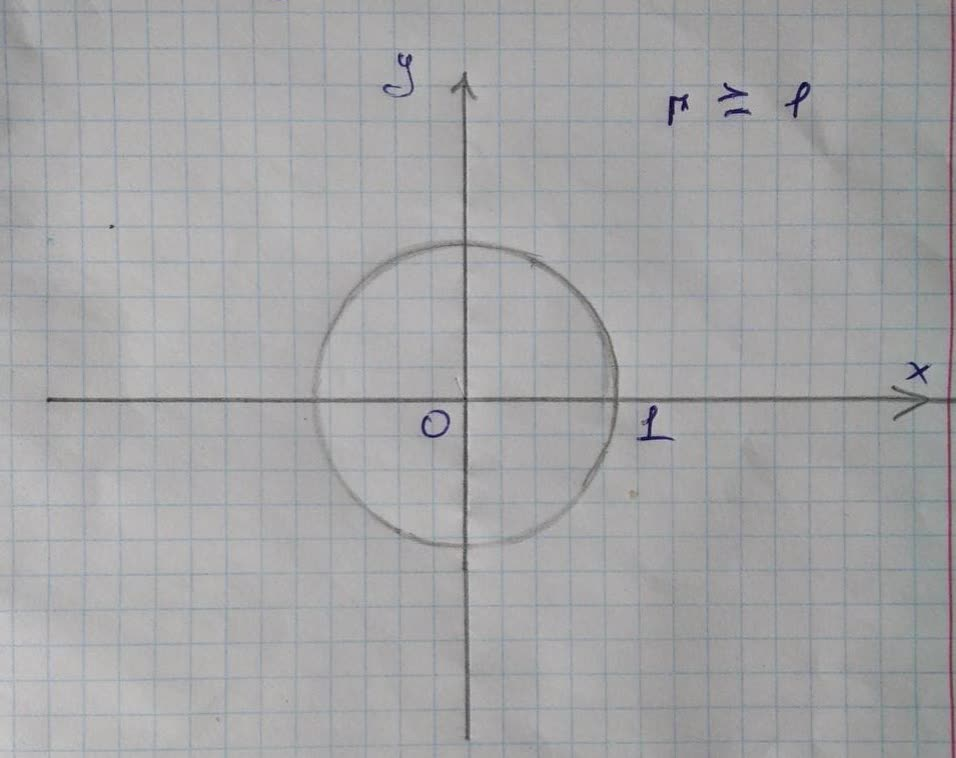Graph the sets of points whose polar coordinates satisfy theambiguit 2021-11-22 Answered
Graph the sets of points whose polar coordinates satisfy the given polar equation.
The given polar equation is written as follows:
$$\displaystyle{r}\geq{1}$$

• Questions are typically answered in as fast as 30 minutes

Solve your problem for the price of one coffee

• Math expert for every subject
• Pay only if we can solve itGeraldine Flores

Description:
Consider the polar coordinates as P (r, 0).
Here, r is the directed distance from origin Oto point Pand O is the directed angle from initial ray to OP.
From the given equation, the range of ris from 1 to infinity.
The 0 varies over any interval of length . $$\displaystyle{2}\pi$$.Therefore, the given polar point traces a circle of radius 1 and centered at origin O (0, 0). The external area of the circle represents the given equation $$r \geq1$$.
From the analysis, draw the sets of points whose polar coordinates satisfy the given polar equation as shown in
Figure 1.